# Civil Engineering - Applied Mechanics

### Exercise :: Applied Mechanics - Section 3

26.

Newtons's Law of Motion is :

 A. Every body continues in its state of rest or of uniform motion, in a straight line, unless it is acted upon by some external force B. The rate of change of momentum is directly proportional to the impressed force, and takes place in the same direction, in which the force acts C. To every action, there is always an equal and opposite reaction D. All the above.

Explanation:

No answer description available for this question. Let us discuss.

27.

The member which does not carry zero force in the structure shown in below figure, is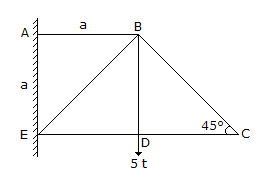A. ED B. DC C. BC D. BD.

Explanation:

No answer description available for this question. Let us discuss.

28.

The equation of motion of a particle starting from rest along a straight line is x = t3 - 3l2 + 5. The ratio of the velocities after 5 sec and 3 sec will be

 A. 2 B. 3 C. 4 D. 5 E. 4.5

Explanation:

No answer description available for this question. Let us discuss.

29.

The moment of inertia of a hollow circular section whose external diameter is 8 cm and internal diameter is 6 cm, about centroidal axis, is

 A. 437.5 cm4 B. 337.5 cm4 C. 237.5 cm4 D. 137.5 cm4 E. 37.5 cm4.

Explanation:

No answer description available for this question. Let us discuss.

30.

The height at which the end of a rope of length l should be tied so that a man pulling at the other end may have the greatest tendency to overturn the pillar, is

 A.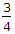l B.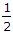C.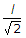D.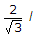E. none of these.